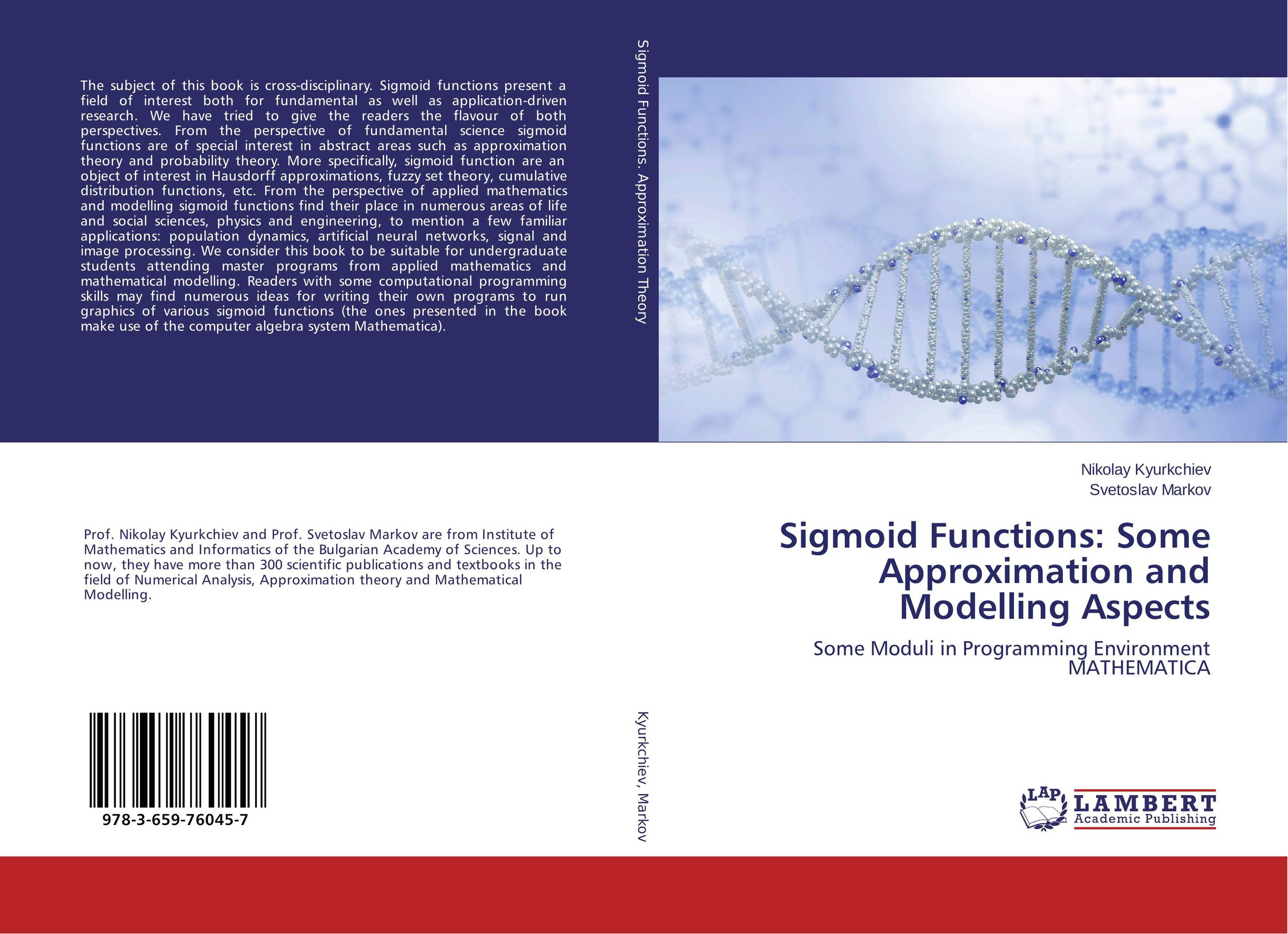# Sigmoid Functions: Some Approximation and Modelling Aspects

###### Аннотация:
The subject of this book is cross-disciplinary. Sigmoid functions present a field of interest both for fundamental as well as application-driven research. We have tried to give the readers the flavour of both perspectives. From the perspective of fundamental science sigmoid functions are of special interest in abstract areas such as approximation theory and probability theory. More specifically, sigmoid function are an object of interest in Hausdorff approximations, fuzzy set theory, cumulative distribution functions, etc. From the perspective of applied mathematics and modelling sigmoid functions find their place in numerous areas of life and social sciences, physics and engineering, to mention a few familiar applications: population dynamics, artificial neural networks, signal and image processing. We consider this book to be suitable for undergraduate students attending master programs from applied mathematics and mathematical modelling. Readers with some computational programming skills may find numerous ideas for writing their own programs to run graphics of various sigmoid functions (the ones presented in the book make use of the computer algebra system Mathematica).
###### Издания произведения:9783659760457

### Рекомендуем

#### Комментарии

Пока нет ни одного комментария
Вы должны войти для того что бы оставлять комментарии